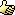A Flash Developer Resource Site

1. ## car movement(friction issue)

Hi. Havnt been in the boards for a long while. Its good to be back.

Anyway, I felt like fooling around with car movement and such, so far ive been able to get the car to move, according to the rotation of the wheels, like a real car.

However, I have a friction issue. The car slides way too much. Its almost as if its on ice. For now Id like to fix the friction to be more like regualr terrain.

You can test it here: Car Movement

and the body of the code can be found here if need be:

Code:
```onClipEvent (load) {
speed = 0;
}
onClipEvent (enterFrame) {

if (Key.isDown(Key.UP)) {
speed += .05;
_rotation += RF_tire._rotation*.09;
}

if (Key.isDown(Key.DOWN)) {
speed -= .05;
_rotation -= RF_tire._rotation*.09;
}

if (Math.abs(speed)>30) {
speed *= .6;
}

if (Key.isDown(Key.RIGHT)) {
if (RF_tire._rotation>=-40 and RF_tire._rotation<=40) {
RF_tire._rotation += 5;
LF_tire._rotation += 5;
//_rotation += 6;
}
}
if (Key.isDown(Key.LEFT)) {
if (RF_tire._rotation>=-40 and RF_tire._rotation<=40) {
RF_tire._rotation -= 5;
LF_tire._rotation -= 5;
//_rotation -= 6;
}
}
if (RF_tire._rotation<=-40) {
RF_tire._rotation += 5;
LF_tire._rotation += 5;
}
if (RF_tire._rotation>=40) {
RF_tire._rotation -= 5;
LF_tire._rotation -= 5;
}

speed *= .98;
x = Math.sin((RF_tire._rotation+_rotation)*(Math.PI/180))*speed;
y = Math.cos((RF_tire._rotation+_rotation)*(Math.PI/180))*speed*-1;
if (!_root.bound.hitTest(_x+x, _y+y, true)) {
_x += x;
_y += y;
} else {
speed *= -.5;
}
}```
I aprreciate any help given to me. I'd like to get nice adjustable friction, for different terrains, if possible.

Thanks again.Reply With Quote

2. The part at the end where you say
speed *= .98;
is the problem.

That means whatever the speed is, it'll only be 2/100ths slower the next frame.

The closer to *= 0 it is, the faster your car will come to a haltBut you may want to make sure that the friction part only happens when none of the movement keys are being pressed... or the car might act like it's in quicksand instead of ice.Reply With Quote

3. Perfect! I knew it had to do with that, i just had to adjust speed increments as well.

Thanks.

Now onto the next, element! Drifting!

Ill be back in this thread with an update soon enough.Reply With Quote

####Posting Permissions

• You may not post new threads
• You may not post replies
• You may not post attachments
• You may not edit your posts
•

 » Home » Movies » Tutorials » Submissions » Board » Links » Reviews » Feedback » Gallery » Fonts » The Lounge » Sound Loops » Sound FX » About FK » Sitemap